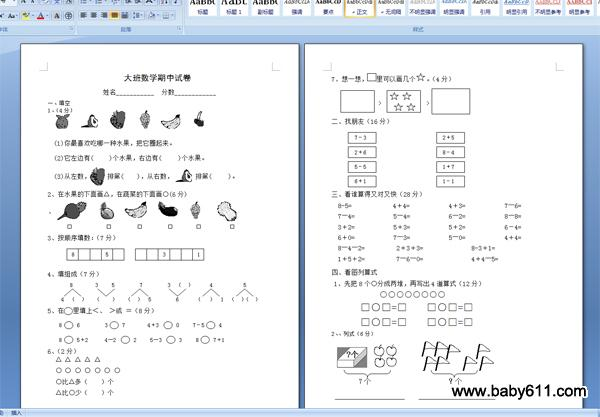幼儿园大班数学期中试卷大班数学期中试卷

姓名___________  分数____________

一、填空

1、（4分）

(1)你最喜欢吃哪一种水果，把它圈起来。

(2)它左边有(     )个水果，右边有(     )个水果。

(3)从左数，  排第(    )，从右数，   排第(     )。

2、在水果的下面画△，在蔬菜的下面画○(6分)

、

□            □         □         □        □          □

3、按顺序填数：（7分）

8531

4、填组成（7分）

8        3     5         7         3  （  ）      4           5

4  （  ）   （    ）   （  ）  5         6      （   ）  1  （  ）（  ）

5、在 里填上＜、 ＞或 ＝（8分）

8   6         3   7        4＋3    0     7－5   4

8   5＋2      4―2    2     5―3    3     8   7＋1

6、（2分）

△ △ △ △ △

○ ○ ○ ○ ○ ○ ○

○比△多（    ）个

△比○少（    ）个

7、想一想， 里可以画几个 。（4分）

＜

>                >

二、找朋友（16分）

三、看谁算得又对又快（28分）

8-5=             4＋4=            4＋3=            7―6=

7―4=            5―4=            6―2=            8―8=

3＋2=            5＋3=            5＋2=            6－4=

6＋0=            7―3=            5―0=            4＋4=

8―4―2=          2＋3＋3=               8-3＋1=

1＋5＋2=          7―6―0=               4＋4―5=

四、看图列算式

1、先把8个○分成两堆，再写出4道算式（12分）

○○○○○○○○

□○□=□      □○□=□

□○□=□      □○□=□

2、、列式（6分）

7个                      ？个

五.思考题（10分）

1+1=2

1+1+1=3

1+1+1+1=4

1+1+1+1+1……（50个）=（    ）

• 幼儿园试卷分类
• 将此试卷分享到：
• 大班试卷最新更新